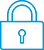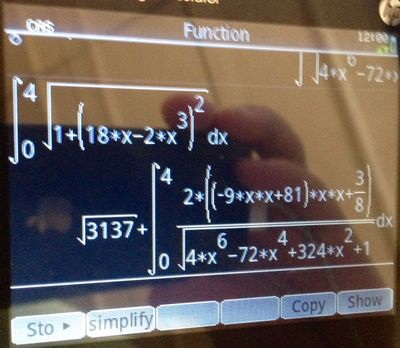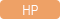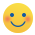cancel
Showing results for
Did you mean:It has been a while since anyone has replied. Simply ask a new question if you would like to start the discussion again.Level 1
11 6 0 0
Message 1 of 4
806
Flag Post

Solved!

# (CAS) Arc Leght Integral returning another integralHP Recommended
HP Prime

Hello!

I`m trying to solve the arc lenght of the function y(x)=2.5+9*xˆ2-0.5xˆ4, between the points x=0 and x=4.

The math to do that is to solve the inegral from 0 to 4 of sqrt(1+(18*x-2*xˆ3)ˆ2) dx

The question is:

When I try to solve this particular integral, the calculator returns me another integral, in a recursive way, as if it can`t fully solve the first integral.Does anybody know how to setup the calculator to be able to solve this kind of integral ?

My CAS setup is currently:

Numeric format : Standard

Simplify: None

Exact [v]

Use sqrt [v]

Principal [v]

Recursive Evaluation: 10

Recursive Replacement: 2

Recursive Function: 20

Epsilon: 1E-12

Probability: 1E-15

Newton: 80

P.S: Wolfram Alpha does solve this kind of integral.

Tags (1)
3 REPLIES 3Level 8
747 744 121 187
Message 2 of 4
Flag PostHP Recommended

Hello,

Luckily, there is no setup to do here!Basically, you are asking the calculator to solve with a perfect, closed form which I'm not certain exists in this case. It runs for a while, and then when it can't make any more progress, returns the modified internal form "as close to the perfect, exact result" as it can. In this case, that is a square root value, and a modified integral.

Press Shift - ENTER to run the shifted ENTER function for an approximation on that result and the decimal answer pops out. That squiggly = sign is the mathematical sign for "approximates to".

Note that if you included a . in your initial entry, the approximate would immediately pop out as the CAS hits that apprixmate number and then continues in a "decimal approximation" form.

sqrt(1.+(18*x-2*xˆ3)ˆ2) <--- note the . after the 1 indicates to the calculator "this is not a perfect, idea of 1 but rather an approximate number that is 1". That is a pretty big difference in mathematics.

If you put a 1. into your original input, it would immediately pop out the decimal. However, just using the Shift ENTER key as needed is generally the simplest way to do it.

TW

Although I work for the HP calculator group as a head developer of the HP Prime, the views and opinions I post here are my own.
Tags (1)Level 9
3,551 3,490 180 541
Message 3 of 4
Flag PostHP Recommended

Hi!, @luiz_aug:

See, this image, for comparison between Wolfram Alpha and HP PRIME (exact and approximate) ....Kind Regards !.
Have a nice day !.HP Recommended